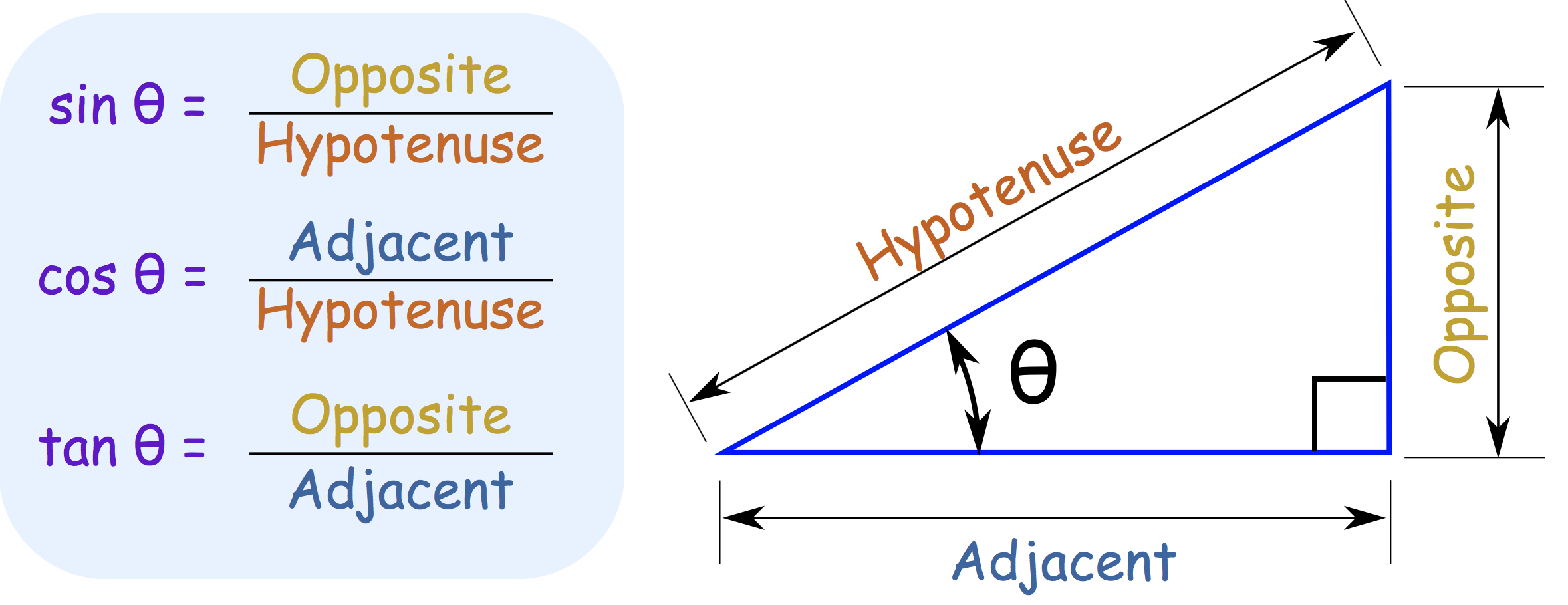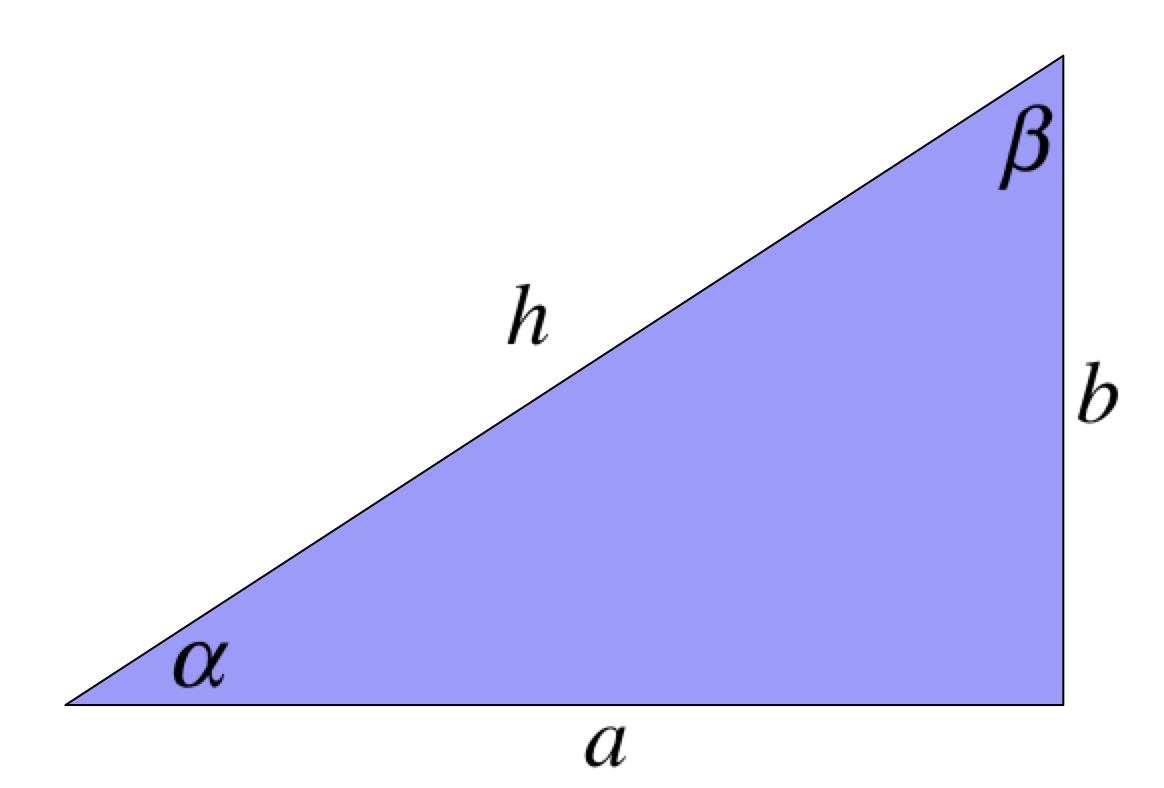# Math Review

Trigonometry

## Introduction

Trigonometry involves using the functions $\cos, \sin, \tan$ and their inverse to calculate lengths and angles of right angle triangles. Any college level physics courses you take will assume knowledge of trigonometry so it is important that you learn at least the very basic.

The three basic trig functions $\cos, \sin, \tan$ are ratio of lengths defined by the following figure:An informal explanation of the names:
• Hypotenuse: the longest side in a right angle triangle
• Adjacent side: the side that touches the angle $\theta$
• Opposite side: the side that is opposite to (and therefore not touching) the angle $\theta$

## Trignometry in Physics

In physics, usually the hypotenuse ($h$) is given and the trig functions are used to find the lengths of the other two sides: \begin{align} \text{adjacent side} &= h \cos \theta \\ \text{opposite side} &= h \sin \theta \\ \end{align}
It is very important to remember that the names "adjacent" and "opposite" depends on which angle you are using. For example, look at the figure below:For this triangle, $a$ is the adjacent side of the angle $\alpha$ (because it touches $\alpha$), but it is the opposite side of the angle $\beta$ (because it does not touch $\beta$). In other words, if you are given $\alpha$, you would calculate the sides by: $$\begin{eqnarray} a &=& \text{adjacent side to }\alpha &=& h \cos \alpha \\ b &=& \text{opposite side to }\alpha &=& h \sin \alpha \end{eqnarray}$$ On the other hand, if you are given $\beta$ then you will have to do this instead: $$\begin{eqnarray} a &=& \text{opposite side to }\beta &=& h \sin \beta \\ b &=& \text{adjacent side to }\beta &=& h \cos \beta \end{eqnarray}$$
The "adjacent side" is not always the horizontal side, nor is the "opposite side" always vertical.

### Simulation - Trigonometry: Sin and Cos (click to hide)

Canvas not supported
Drag on the triangle to change its orientation.
Use the sliders to change the angle and the length.
The "Switch Angle" shows how to use the other angle to perform the calculation.

## The Inverse Trig Functions

The inverse of the trig functions are usually denote as $\cos^{-1}, \sin^{-1}$ and $\tan^{-1}$. Formally they are called the arccosine, arcsine, and arctangent functions.